# 基础评分和排名

## 直接评分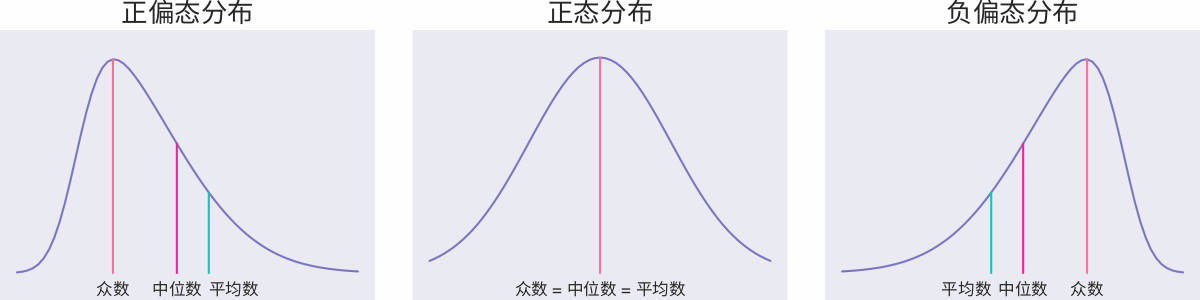# 考虑时间的评分和排名

## Delicious## Hacker News

Hacker News 是一个可以发布帖子的网络社区，每个帖子前面有一个向上的三角形，如果用户觉得这个内容好，点击一下即可投票。根据得票数，系统自动统计出热门文章排行榜。Hacker News 使用分数计算公式如下：

$$Score = \dfrac{P - 1}{\left(T + 2\right)^G} \label{eq:hacker-news}$$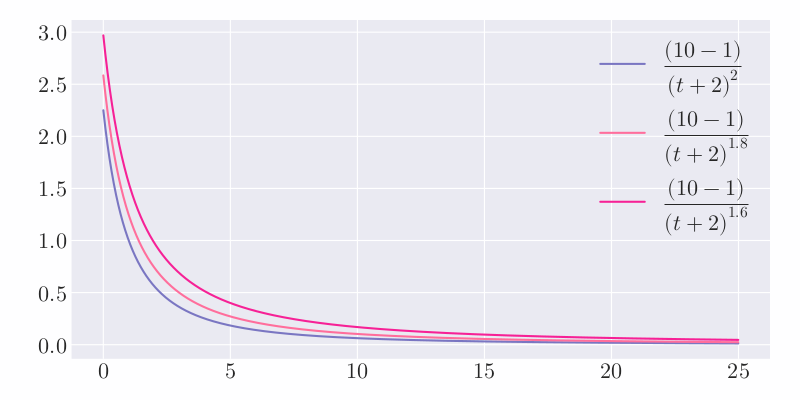## RedditReddit 关于计算分数的代码可以简要总结如下：

from datetime import datetime, timedelta
from math import log

epoch = datetime(1970, 1, 1)

def epoch_seconds(date):
td = date - epoch
return td.days * 86400 + td.seconds + (float(td.microseconds) / 1000000)

def score(ups, downs):
return ups - downs

def hot(ups, downs, date):
s = score(ups, downs)
order = log(max(abs(s), 1), 10)
sign = 1 if s > 0 else -1 if s < 0 else 0
seconds = epoch_seconds(date) - 1134028003
return round(order + sign * seconds / 45000, 7)


1. 计算赞成票和反对票的差值，即： $$s = ups - downs$$
2. 利用如下公式计算中间分数，即： $$order = \log_{10} \max\left(\left|s\right|, 1\right)$$ 其中，取 $\left|s\right|$ 和 $1$ 的最大值是为了避免当 $s = 0$ 时，无法计算 $\log_{10}{\left|s\right|}$。赞成票与反对票差值越大，得分越高。取以 $10$ 为底的对数，表示当 $s = 10$ 时，这部分为 $1$，只有 $s = 100$ 时才为 $2$，这样设置是为了减缓差值增加对总分的影响程度。
3. 确定分数的方向，即： $$sign = \begin{cases} 1 & \text{如果} \ s > 0 \\ 0 & \text{如果} \ s = 0 \\ -1 & \text{如果} \ s < 0 \end{cases}$$
4. 计算发贴时间距离 2005 年 12 月 8 日 7:46:43（Reddit 的成立时间？）的秒数，即： $$seconds = \text{timestamp}\left(date\right) - 1134028003$$
5. 计算最终分数，即： $$score = order + sign \times \dfrac{seconds}{45000}$$ 将时间除以 $45000$ 秒（即 12.5 个小时），也就是说当前天的帖子会比昨天的帖子多约 $2$ 分。如果昨天的帖子想要保持住之前的排名，则 $s$ 值需要增加 $100$ 倍才可以。

Reddit 评分排名算法决定了 Reddit 是一个符合大众口味的社区，而不是一个适合展示激进想法的地方。因为评分中使用的是赞成票和反对票的差值，也就是说在其他条件相同的情况下，帖子 A 有 1 票赞成，0 票反对；帖子 B 有 1000 票赞成，1000 票反对，但讨论火热的帖子 B 的得分却比 帖子 A 要少。

## Stack Overflow

Stack Overflow 是世界排名第一的程序员问答社区。用户可以在上面提出各种关于编程的问题，等待别人回答；可以对问题进行投票（赞成票或反对票），表示这个问题是不是有价值；也可以对这个回答投票（赞成票或反对票），表示这个回答是不是有价值。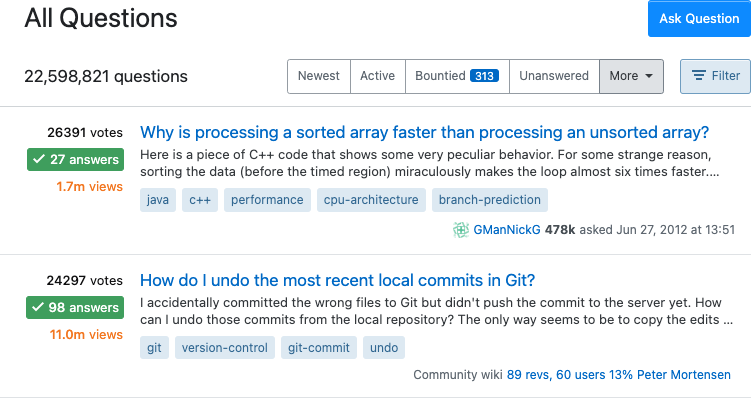$$\dfrac{4 \times \log_{10}{Q_{views}} + \dfrac{Q_{answers} \times Q_{score}}{5} + \sum \left(A_{scores}\right)}{\left(\left(Q_{age} + 1\right) - \left(\dfrac{Q_{age} - Q_{updated}}{2}\right)\right)^{1.5}}$$

1. $4 \times \log_{10}{Q_{views}}$ 表示问题的浏览次数越多，得分越高，同时利用 $\log_{10}$ 减缓了随着浏览量增大导致得分变高的程度。
2. $\dfrac{Q_{answers} \times Q_{score}}{5}$ 表示问题的得分（赞成票和反对票之差）越高，回答的数量越多，分数越高。采用乘积的形式意味着即使问题本身的分数再高，没有人回答的问题也算不上热门问题。
3. $\sum \left(A_{scores}\right)$ 表示问题回答的总分数。回答总分采用了简单的加和，但实际上一个正确的回答要胜过多个无用的回答，简答的加和无法很好的区分这两种不同的情况。
4. $\left(\left(Q_{age} + 1\right) - \left(\dfrac{Q_{age} - Q_{updated}}{2}\right)\right)^{1.5}$ 可以改写为 $\left(\dfrac{Q_{age}}{2} + \dfrac{Q_{updated}}{2} + 1\right)^{1.5}$$Q_{age}$$Q_{updated}$ 分别表示问题和最近一次回答的时间（单位为小时），也就是说问题时间越久远，最近一次回答时间约久远，分母就会越大，从而得分就会越小。

Stack Overflow 的评分排名算法考虑了参与程度（问题浏览次数和回答次数）、质量（问题分数和回答分数）、时间（问题时间和最近一次回答时间）等多个维度。

# 不考虑时间的评分和排名

## 威尔逊区间算法

$$\text{评分} = \text{赞成票} - \text{反对票}$$

$$\text{评分} = \dfrac{\text{赞成票}}{\text{赞成票} + \text{反对票}}$$

1. 每个用户的投票都是独立事件。
2. 用户只有两个选择，要么投赞成票，要么投反对票。
3. 如果投票总人数为 $n$，其中赞成票为 $k$，则赞成票的比例 $p = \dfrac{k}{n}$

1. 计算每个项目的好评率。
2. 计算每个好评率的置信区间。
3. 根据置信区间的下限值进行排名。

$$\frac{1}{1+\frac{z^{2}}{n}}\left(\hat{p}+\frac{z^{2}}{2 n}\right) \pm \frac{z}{1+\frac{z^{2}}{n}} \sqrt{\frac{\hat{p}(1-\hat{p})}{n}+\frac{z^{2}}{4 n^{2}}}$$

## 贝叶斯平均算法

$$WR = \dfrac{v}{v + m} R + \dfrac{m}{v + m} C$$

$$\bar{x}=\frac{C m+\sum_{i=1}^{n} x_{i}}{C+n}$$

# 比赛评分和排名

## Kaggle 积分

Kaggle 是一个数据建模和数据分析竞赛平台。企业和研究者可在其上发布数据，统计学者和数据挖掘专家可在其上进行竞赛以产生最好的模型。用户以团队形式参加 Kaggle 的比赛，团队可以仅包含自己一人，根据在每场比赛中的排名不断获取积分，用做 Kaggle 网站中的最终排名

$$\left[\dfrac{100000}{N_{\text {teammates }}}\right]\left[\text{Rank}^{-0.75}\right]\left[\log _{10}\left(N_{\text {teams }}\right)\right]\left[\dfrac{2 \text { years - time }}{2 \text { years }}\right]$$

$$\left[\dfrac{100000}{\sqrt{N_{\text {teammates }}}}\right]\left[\text{Rank}^{-0.75}\right]\left[\log _{10}\left(1+\log _{10}\left(N_{\text {teams }}\right)\right)\right]\left[e^{-t / 500}\right]$$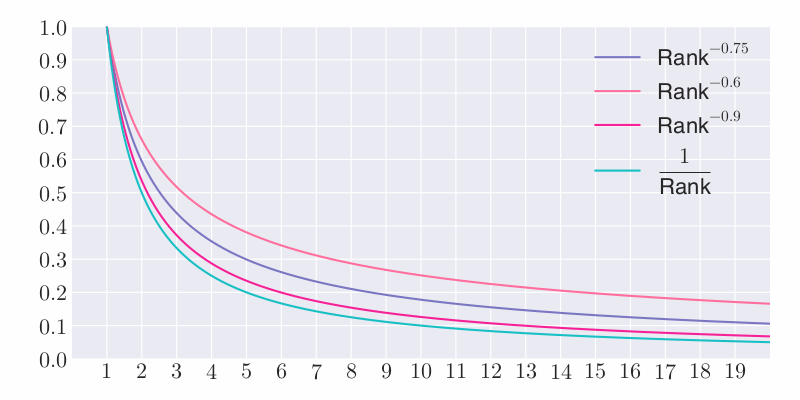\begin{equation} \begin{aligned} \log_{10} \left(100\right) &= 2 \\ \log_{10} \left(1000\right) &= 3 \end{aligned} \end{equation}

\begin{equation} \begin{aligned} \log_{10} \left(\log_{10} \left(100\right) + 1\right) &\approx 0.47 \\ \log_{10} \left(\log_{10} \left(1000\right) + 1\right) &\approx 0.6 \end{aligned} \end{equation}

## Elo 评分系统

Elo 评分系统（Elo Rating System）是由匈牙利裔美国物理学家 Arpad Elo 创建的一个衡量各类对弈活动水平的评价方法，是当今对弈水平评估公认的权威标准，且被广泛用于国际象棋、围棋、足球、篮球等运动。网络游戏的竞技对战系统也常采用此评分系统。

Elo 评分系统是基于统计学的一个评估棋手水平的方法。Elo 模型原先采用正态分布，但实践显明棋手的表现并非正态分布，所以现在的评分计分系统通常使用的是逻辑分布。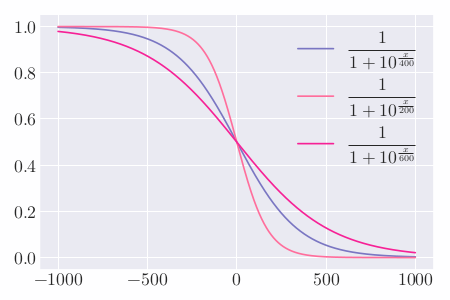$$E_{A}=\frac{1}{1+10^{\left(R_{B}-R_{A}\right) / 400}}$$

$$E_{B}=\frac{1}{1+10^{\left(R_{A}-R_{B}\right) / 400}}$$

$$R_{A}^{\prime} = R_{A} + K\left(S_{A}-E_{A}\right)$$

## Glicko 评分系统

Glicko 评分系统（Glicko Rating System）及 Glicko-2 评分系统（Glicko-2 Rating System）是评估选手在比赛中（如国际象棋及围棋）的技术能力方法之一。此方法由马克·格利克曼发明，原为国际象棋评分系统打造，后作为评分评分系统的改进版本广泛应用 1

Elo 评分系统的问题在于无法确定选手评分的可信度，而 Glicko 评分系统正是针对此进行改进。假设两名评分均为 1700 的选手 A 和 B 在进行一场对战后 A 获得胜利，在美国国际象棋联赛的 Elo 评分系统下，A 选手评分将增长 16，对应的 B 选手评分将下降 16。但是假如 A 选手是已经很久没玩，但 B 选手每周都会玩，那么在上述情况下 A 选手的 1700 评分并不能十分可信地用于评定其实力，而 B 选手的 1700 评分则更为可信。

Glicko 算法的主要贡献是“评分可靠性”（Ratings Reliability），即评分偏差（Ratings Deviation）。若选手没有评分，则其评分通常被设为 1500，评分偏差为 350。新的评分偏差（$RD$）可使用旧的评分偏差（$RD_0$）计算：

$$RD = \min \left(\sqrt{RD_0^2 + c^2 t}, 350\right)$$

$$r=r_{0}+\frac{q}{\frac{1}{R D^{2}}+\frac{1}{d^{2}}} \sum_{i=1}^{m} g\left(R D_{i}\right)\left(s_{i}-E\left(s \mid r_{0}, r_{i}, R D_{i}\right)\right)$$

\begin{equation*} \begin{aligned} & g\left(R D_{i}\right) = \frac{1}{\sqrt{1+\frac{3 q^{2}\left(R D_{i}^{2}\right)}{\pi^{2}}}} \\ & E\left(s \mid r, r_{i}, R D_{i}\right) = \frac{1}{1+10\left(\frac{g\left(R D_{i}\right)\left(r_{0}-r_{i}\right)}{-400}\right)} \\ & q = \frac{\ln (10)}{400}=0.00575646273 \\ & d^{2} = \frac{1}{q^{2} \sum_{i=1}^{m}\left(g\left(R D_{i}\right)\right)^{2} E\left(s \mid r_{0}, r_{i}, R D_{i}\right)\left(1-E\left(s \mid r_{0}, r_{i}, R D_{i}\right)\right)} \end{aligned} \end{equation*}

$r_i$ 表示选手个人评分，$s_i$ 表示每场比赛后的结果。胜利为 $1$，平局为 $1 / 2$，失败为 $0$

$$R D^{\prime}=\sqrt{\left(\frac{1}{R D^{2}}+\frac{1}{d^{2}}\right)^{-1}}$$

## Glicko-2 评分系统

Glicko-2 算法与原始 Glicko 算法类似，增加了一个评分波动率 $\sigma$，它根据玩家表现的不稳定程度来衡量玩家评分的预期波动程度。例如：当一名球员的表现保持稳定时，他们的评分波动性会很低，如果他们在这段稳定期之后取得了异常强劲的成绩，那么他们的评分波动性就会增加 1

Glicko-2 算法的简要步骤如下：

### 计算辅助量

\begin{aligned} v &= \left[\sum_{j=1}^{m} g\left(\phi_{j}\right)^{2} E\left(\mu, \mu_{j}, \phi_{j}\right)\left\{s_{j}-E\left(\mu, \mu_{j}, \phi_{j}\right)\right\}\right]^{-1} \\ \Delta &= v \sum_{j=1}^{m} g\left(\phi_{j}\right)\left\{s_{j}-E\left(\mu, \mu_{j}, \phi_{j}\right)\right\} \end{aligned}

\begin{equation*} \begin{aligned} &g(\phi)=\frac{1}{\sqrt{1+3 \phi^{2} / \pi^{2}}}, \\ &E\left(\mu, \mu_{j}, \phi_{j}\right)=\frac{1}{1+\exp \left\{-g\left(\phi_{j}\right)\left(\mu-\mu_{j}\right)\right\}} \end{aligned} \end{equation*}

### 确定新的评分波动率

$$f(x)=\frac{1}{2} \frac{e^{x}\left(\Delta^{2}-\phi^{2}-v^{2}-e^{x}\right)}{\left(\phi^{2}+v+e^{x}\right)^{2}}-\frac{x-\ln \left(\sigma^{2}\right)}{\tau^{2}}$$

$$\sigma' = e^{\frac{A}{2}}$$

### 确定新的评分偏差和评分

$$\phi^{\prime} = \dfrac{1}{\sqrt{\dfrac{1}{\phi^{2}+\sigma^{\prime 2}}+\dfrac{1}{v}}}$$

$$\mu^{\prime} = \mu+\phi^{\prime 2} \sum_{j=1}^{m} g\left(\phi_{j}\right)\left\{s_{j}-E\left(\mu, \mu_{j}, \phi_{j}\right)\right\}$$

## TrueSkill 评分系统

TrueSkill 评分系统是基于贝叶斯推断的评分系统，由微软研究院开发以代替传统 Elo 评分系统，并成功应用于 Xbox Live 自动匹配系统。TrueSkill 评分系统是 Glicko 评分系统的衍伸，主要用于多人游戏中。TrueSkill 评分系统考虑到了个别玩家水平的不确定性，综合考虑了各玩家的胜率和可能的水平涨落。当各玩家进行了更多的游戏后，即使个别玩家的胜率不变，系统也会因为对个别玩家的水平更加了解而改变对玩家的评分 2

1. 一个包含跟踪所有玩家比赛结果，记录玩家能力的模块。
2. 一个对比赛成员进行配对的模块。
3. 一个公布比赛中各成员能力的模块。

### 能力计算和更新

TrueSkill 评分系统是针对玩家能力进行设计的，以克服现有排名系统的局限性，确保比赛双方的公平性，可以在联赛中作为排名系统使用。TrueSkill 评分系统假设玩家的水平可以用一个正态分布来表示，而正态分布可以用两个参数：平均值和方差来完全描述。设 Rank 值为 $R$，代表玩家水平的正态分布的两个参数平均值和方差分别为 $\mu$$\sigma$，则系统对玩家的评分即 Rank 值为：

$$R = \mu - k \times \sigma$$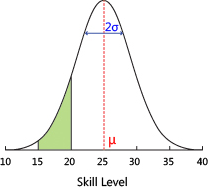Alice 1 25 8.3 36.771 5.749
Bob 2 25 8.3 32.242 5.133
Chris 3 25 8.3 29.074 4.943
Darren 4 25 8.3 26.322 4.874
Eve 5 25 8.3 23.678 4.874
Fabien 6 25 8.3 20.926 4.943
George 7 25 8.3 17.758 5.133
Hillary 8 25 8.3 13.229 5.749

\begin{aligned} &\mu_{\text {winner }} \longleftarrow \mu_{\text {winner }}+\frac{\sigma_{\text {winner }}^{2}}{c} * v\left(\frac{\mu_{\text {winner }}-\mu_{\text {loser }}}{c}, \frac{\varepsilon}{c}\right) \\ &\mu_{\text {loser }} \longleftarrow \mu_{\text {loser }}-\frac{\sigma_{\text {loser }}^{2}}{c} * v\left(\frac{\mu_{\text {winner }}-\mu_{\text {loser }}}{c}, \frac{\varepsilon}{c}\right) \\ &\sigma_{\text {winner }}^{2} \longleftarrow \sigma_{\text {uninner }}^{2} *\left[1-\frac{\sigma_{\text {winner }}^{2}}{c} * w\left(\frac{\mu_{\text {winner }}-\mu_{\text {loser }}}{c}, \frac{\varepsilon}{c}\right)\right. \\ &\sigma_{\text {loser }}^{2} \longleftarrow \sigma_{\text {loser }}^{2} *\left[1-\frac{\sigma_{\text {loser }}^{2}}{c} * w\left(\frac{\mu_{\text {winner }}-\mu_{\text {loser }}}{c}, \frac{\varepsilon}{c}\right)\right. \\ &c^{2}=2 \beta^{2}+\sigma_{\text {winner }}^{2}+\sigma_{\text {loser }}^{2} \end{aligned}

### 对手匹配

$$e^{-\frac{\left(\mu_{A}-\mu_{B}\right)^{2}}{2 c^{2}}} \sqrt{\frac{2 \beta^{2}}{c^{2}}}$$

$c$ 的值由如下公式给出：

$$c^{2}=2 \beta^{2}+\mu_{A}^{2}+\mu_{B}^{2}$$

$$R=25-3 \frac{25}{3}=0$$

1. 适用于复杂的组队形式，更具一般性。
2. 有更完善的建模体系，容易扩展。
3. 继承了贝叶斯建模的优点，如模型选择等。